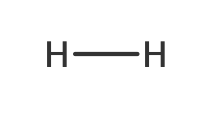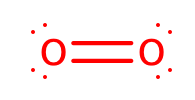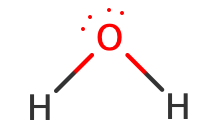# Problem: If hydrogen were used as a fuel, it could be burned according to the following reaction: H2(g)+1/2 O2(g)→H2O(g).A) Use average bond energies to calculate ΔHrxn for this reaction. (Answer for this part is -243 kJ/mol but I was unable to get this number when I tried solving it.)B) Use average bond energies to calculate ΔHrxn for the combustion of methane (CH4).C) Which fuel yields more energy per mole: hydrogen or methane?D) Per gram: hydrogen or methane?

###### FREE Expert Solution

To calculate the ΔH°rxn using bond energies, we’re going to use the following equation:

$\overline{){\mathbf{∆}}{\mathbf{H}}{{\mathbf{°}}}_{{\mathbf{rxn}}}{\mathbf{=}}{\mathbf{∆}}{\mathbf{H}}{{\mathbf{°}}}_{{\mathbf{reactants}}}{\mathbf{-}}{\mathbf{∆}}{\mathbf{H}}{{\mathbf{°}}}_{{\mathbf{products}}}}$

Part A.

Balanced Reaction: H2(g) + 1/2 O2(g) → H2O(g)

*always make sure that the given reaction is balanced

Draw the structure of each compound.

Reactants:

H2(g):O2(g):Products:

H2O:83% (135 ratings)###### Problem Details

If hydrogen were used as a fuel, it could be burned according to the following reaction: H2(g)+1/2 O2(g)→H2O(g).

A) Use average bond energies to calculate ΔHrxn for this reaction. (Answer for this part is -243 kJ/mol but I was unable to get this number when I tried solving it.)

B) Use average bond energies to calculate ΔHrxn for the combustion of methane (CH4).

C) Which fuel yields more energy per mole: hydrogen or methane?

D) Per gram: hydrogen or methane?